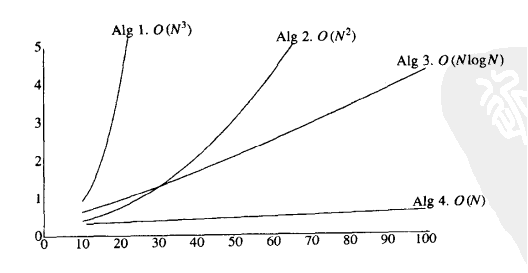### 数学基础

#### 定义

• 如果存在正常数 c 和 n，使得当 N>=n 时，T(N)<=cf(N)，则记为 T(N)=O(f(n))。
• 如果存在正常数 c 和 n，使得当 N<=n 时，T(N)>=cg(N)，则记为 T(N)=Ω(g(n))。

### 最大子序问题

#### 运行时间计算#### 一般法则

1. for 循环
一次 for 循环运行的时间至多是该 for 循环内语句的运行时间乘以迭代次数。
2. 嵌套 for 循环
从里向外分析这些 for 循环，例如：
``````for (i = 0; i < N; i++)
for (j = 0; j < N; j++)
k++;
``````

``````for (i = 0; i < N; i++)
k++;
for (i = 0; i < N; i++)
for (j = 0; j < N; j++)
k--;
``````
1. if/else 语句
``````if (condition)
S1;
else
S2;
``````

#### 最大子序和的算法分析

``````int main()
{
int a = {-2, 11, -4, 13, -5, -2}, N = 6;
printf("%d\n", maxSubsquenceSum_X(a, N));
return 0;
}
``````
1. 算法 1
``````#include<stdio.h>
int maxSubsquenceSum1(const int a[], int N)
{
int thisSum, maxSum, i, j, k;
maxSum = 0;

for(i = 0; i < N; i++)
{
for(j = i; j < N; j++)
{
thisSum = 0;
for(k = i; k <= j; k++)
{
thisSum += a[k];
if(thisSum > maxSum)
{
maxSum = thisSum;
}
}
}
}

return maxSum;
}
``````

1. 算法 2
``````int maxSubsquenceSum2(const int a[], int N)
{
int thisSum, maxSum, i, j;

maxSum = 0;
for(i = 0; i < N; i++)
{
thisSum = 0;
for(j = i; j < N; j++)
{
thisSum += a[j];
if(thisSum > maxSum)
{
maxSum = thisSum;
}
}
}

return maxSum;
}
``````

1. 算法 3
``````int maxSubsquenceSum4(const int a[], int N)
{
int thisSum, maxSum, j;

thisSum = maxSum = 0;
for(j = 0; j < N; j++)
{
thisSum += a[j];
if(thisSum > maxSum)
{
maxSum = thisSum;
}
else if(thisSum < 0)
{
thisSum = 0;
}
}

return maxSum;
}
``````